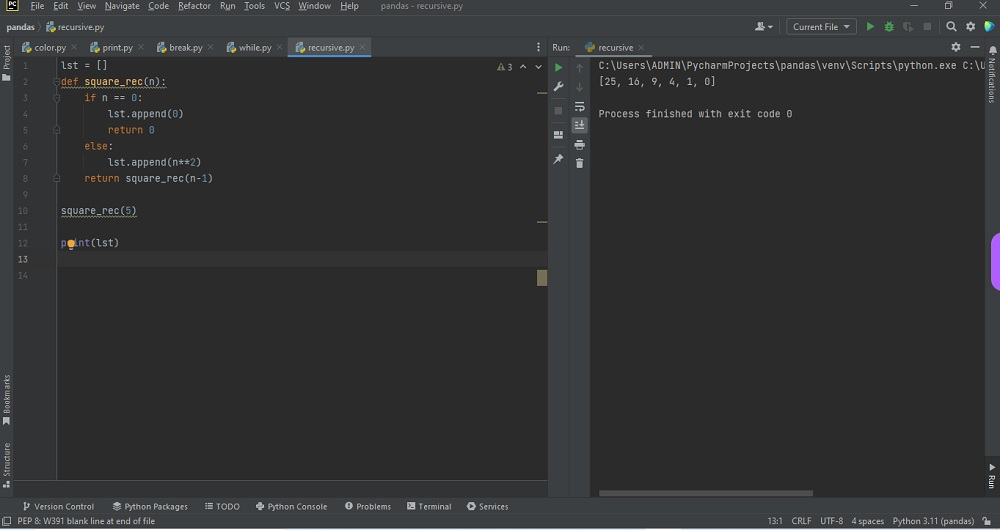# Recursive function pythonby vsasikalabeUpdated: Feb 22, 2023Guide Kit

A recursive function is a function defined as a self-referential expression. This function is used to continue calling itself and repeat its behavior until some condition is met to return a result. Using this operator, Using 2 as the exponent, you can find a number's square. Suppose conditions are used in the Recursive function. When n=0, the result returns 0. Otherwise, it appends the square value.

The three ways are to square a number as follows:

1. **, the power operator
2. the in-built pow() function
3. the math.pow() function from the math module

** is called the power operator. Here is the syntax:

number ** exponent

To define something in terms of itself Recursion process is used. Where we place two parallel mirrors, any object in between would be reflected recursively is a physical word example. It will recursively call itself by reducing the number when we call this function with a positive integer. To stop the recursion, or else the function calls itself infinitely, every recursive function must have a base condition. The Python interpreter is used to limit the recursion depths to help avoid infinite recursions, resulting in stack overflows.

• The code looks clean and elegant.
• Sequence generation is easier with recursion.
• A complex task can be divided into simpler sub-problems using recursion.

• Sometimes the logic takes effort to follow.
• Recursive functions are hard to debug.
• Recursive calls are expensive (inefficient)
• It takes up a lot of memory and time.

Here is an example of how to do a Recursive function using Python:Fig : Preview of the output that you will get on running this code from your IDE.

### Instructions

Follow the steps carefully to get the output easily.

1. Copy the snippet using the 'copy' and paste it in your IDE.
2. Add required dependencies and import them in Python file.
3. Run the file to generate the output.

I hope you found this useful.

I found this code snippet by searching for ' Recursive function python'in kandi. You can try any such use case!

### Environment Tested

I tested this solution in the following versions. Be mindful of changes when working with other versions.

1. The solution is created in PyCharm 2021.3.
2. The solution is tested on Python 3.9.7.

Using this solution, we are able to do Recursive function in python with simple steps. This process also facilities an easy way to use, hassle-free method to create a hands-on working version of code which would help us to do Recursive function in python.

### Support

1. For any support on kandi solution kits, please use the chat
2. For further learning resources, visit the Open Weaver Community learning page.